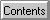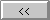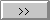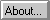# Satellite Antenna Pattern

Pattern Loss
Most satellites of interest to radio amateurs have relatively low gain antennas, since the object is to obtain a large coverage (the whole footprint), and the cost of steering a satellite with a high gain antenna is prohibitive. For purposes of gain calculations, the antenna gain when correctly pointed is therefore assumed to be unity (0dB).
However, the satellite antennas may not be pointed directly at the ground station (observer). The deviation from a direct line is called the squint angle and depends on the spacecraft attitude. This introduces a pattern loss, since the antenna efficiency is generally less when the antenna is not correctly pointed. In WinOrbit, the pattern loss is calculated from the formula:
Pattern Loss(dB) = 20 log (|cos(squint)|)
for angles less than 90 degrees. This is only approximate, of course, since each antenna type has its own characteristic pattern. In any case, the computed pattern loss is limited to -40dB, since even a very good directional antenna is likely to have leakage larger than this. Likewise, for angles larger than 90, the loss is set to -40 dB.

Converted by Winhelp to Web